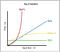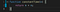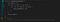# Big O— For Beginners

If you get into Web development, Software Development or anything to do with writing code, you well eventually come across something called Big O Notation. It won’t make much sense in the beginning of your journey but when you start learning algorithms, applying for jobs or preparing for interviews, you’re going to need some knowledge of Big O.

# What is Big O Notation?

Simply put, Big O is used to help us estimate the Run-time Complexity of a function and how it scales when the input increases. Meaning that if we give a function an Array with 10 items vs giving that same function an Array of 10,000 items, we want to understand the performance of that function with regards to input. When we see O(n), we are saying “Order of N”, where N is the input count. We will go over just a couple Big O complexities: O(1) — Constant, O(N) — Linear, O(n²) — Quadratic.Fig 1. Big O run times

## Constant Time — O(1):Constant Time

The code above illustrates O(1) or Constant Time because it doesn’t matter how large N is, the quantity of operations this function is performing stays the same. So if n = 100 or n= 100000, we are still only doing one operation (n + n) and returning that value. Constant is almost always the ideal run time complexity for algorithms, but not always achievable. To see what Constant time looks like on a graph, see Figure 1, green line.

## Linear Time — O(n):

The code above illustrates Linear Time because the quantity of operations is directly proportional to n length. If n = 10 or n = 100,000, we will loop through 10 or 100,000 times respectively. Our quantity of operations increases as our n input increases and will result in run times increasing. The blue line in this graph reflects Linear time.

## Quadratic Time — O(n^2):

Don’t be alarmed by the word “Quadratic”, after all the word “quadratic” comes from quadratum, the Latin word for square.Quadratic Time

The code above illustrates Quadratic Time — O(n²) because the length of n makes the quantity of operations increase by n² amount. So in the example above we have n length = 3 so 3² is equal to 9(operations), if n length was 10 we would have 10² or 100 operations, if n length was 1000 we would have 1000² or 1,000,000 operations. As you can see Quadratic Time can slow down quickly as n increases.The run-time of Quadratic Time complexities in Figure 1 is the red line.

This is an overly simplified version of Big O notation but hopefully this will ease your anxiety a little. Big O can get complicating quick, but just like anything else, it takes time and practice!